• 从构造大气湍流模型，运动模糊模型，gauss噪声模型开始，利用逆滤波和半径受限逆滤波还有维纳滤波处理，分成多个脚本函数文件易于调试，对比效果明显
• 今天小编就为大家分享一篇python实现逆滤波与维纳滤波示例，具有很好的参考价值，希望对大家有所帮助。一起跟随小编过来看看吧
• 压缩包里面有6个.m文件, blurring.m用于给图像增加抖动效果 inverseFilter.m是不考虑噪声的逆滤波 inverseFilterWithNoise.m是考虑噪声的逆滤波 wienerFiltering.m是维纳滤波 另外两个是辅助函数维纳滤波
• 图像处理课程作业 可直接运行 附带评价指标：PSNR MSE ...并与逆滤波的方法进行对比。最后采用PSNR和MSE对维纳滤波的结果进行评价。 由于存在取整误差，就算去掉高斯噪声，逆滤波仍然难以完全还原原始图像。
• 图像处理 逆滤波处理 C/C++语言实现..........................
• GUI，用于应用逆滤波、截断逆滤波、维纳滤波和约束最小二乘滤波“metrics.m”——一个用户定义的函数来计算 PSNR 和 SSIM。 在“ImageRestoration.m”中被调用 “Butter_LPF.m” - 一个用户定义的函数，用于在具有...
• 对图像进行模糊处理，然后加白高斯噪声，用逆滤波和维纳滤波恢复图像滤波
• 对一副数字图像模拟出运动模糊效果并采用维纳滤波；模拟出大气湍流效果并采用逆滤波。MATLAB r2013a。
• 图像逆滤波与维纳滤波的matlab代码。包括测试图像与代码处理结果图。数字图像处理教材例子的复现。
• DFT的matlab源代码逆滤波 使用逆滤波进行图像还原 full_inverse.py 全逆滤波器 trunc_inverse.py 截断逆滤波器 wiener_filter.py 维纳过滤器 cls_constant_y.py 常数Y项约束的最小二乘 cls_iterative_y.py 用迭代...
• 生成一幅带噪声的运动模糊图像，并采用逆滤波或者维纳滤波进行恢复。
• 此代码提供了一个matlab程序来实现By R.C.Gonzalez中描述的反滤波。 该程序基于500 * 500像素的图像，受大气湍流影响，其退化模型为高斯或高斯。 在程序中可以改变两个参数，该参数是H（i，j）的“exp”表达式中的第...matlab
• 这是用matlab实现图像复原的程序，包括逆滤波、维纳滤波、约束最小二乘方滤波。
• matlab逆滤波改由opencv实现，测试可用opencv 逆滤波
• 逆滤波

千次阅读 2018-10-17 16:19:53
1.逆滤波的问题点 &amp;nbsp; &amp;nbsp; &amp;nbsp; 图像的老化，可以视为以下这样的一个过程。一个是退化函数的影响（致使图片模糊，褪色等），一个可加性噪声的影响。 用算式表示为&amp;nbsp; ...

1.逆滤波的问题点

图像的老化，可以视为以下这样的一个过程。一个是退化函数的影响（致使图片模糊，褪色等），一个可加性噪声的影响。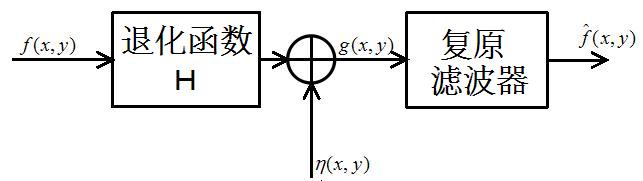用算式表示为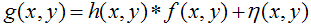前几篇博文，主要是介绍可加性噪声的去除。本博文，主要介绍图像的逆滤波，即退化函数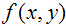的去除。然而，逆滤波在空间域内的处理是很不方便的。
简单的来考虑，加法的逆运算是减法，乘法的逆运算的除法，微分的逆运算是积分(严密一点说是不定积分)。那么就可以得到一个简单的结论了，要出去卷积的话，肯定需要用到卷积的逆运算。卷积的逆运算是---------反卷积，额，好像是一个理所应当的名字。 我们建立了一个关于卷积的直观认识，将信号反转与滤波器系数求积和。那么，反卷积是一种什么样的运算呢？或者具体的来讲，反卷积的空间运算表现形式是什么样的？这样的考虑其实是多余的，或者说，不用考虑的那么复杂。
在之前的博文中([数字图像处理]频域滤波(1)--基础与低通滤波器)，我们得到这样的一个重要的结论。空间域内的卷积，其实就是频域内的乘积。那么这么考虑，就非常简单了，频域内的逆滤波运算，其实就是做除法。我们通过傅里叶变换，可以得到如下一个频域内的老化模型。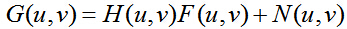这样一个表达式内，没有了卷积运算，是一个很简单的四则运算。那么，所谓的去卷积或者逆滤波，就是将退化函数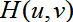去除的过程。这样看来的话，直接做除法就可以了，如下所示。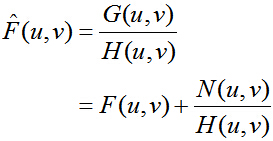按照教材上的说法，这个表达式很有趣（哪里有趣了？）。首先，必须知道精确的退化函数。其次，如果退化函数含有0值或者极小值的话，会使得噪声项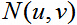变得极大。
综上所述，其实逆滤波的问题点有两个：、
1.退化函数的推测。
2.尽可能的不让噪声项影响画质。

2.两个退化函数的模型

2.1 大气湍流模型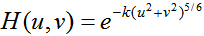这个模型很简单，与高斯LPF很相似。伴随着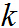值的增大，得到的图像越来越模糊。以下是这个模型执行的结果。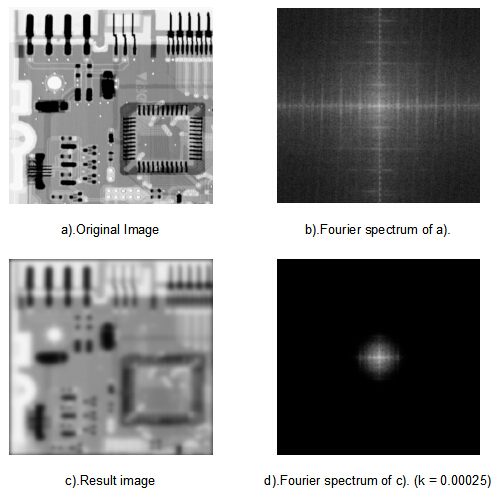从表示式上可以看出，这个模型是不会有0值的，不过，这个模型与低通滤波器很相似，阻带的值都是极小的。这可能会使得图像的直接逆滤波失败。这个之后再说。

2.2 运动模糊模型

这个模型其实在Photoshop上也有一个同名的滤镜。详细的推倒我就不做了，这个模型的表达式如下所示。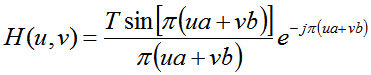这里有几个参数，说明一下。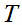表示曝光时间，这里的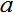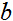表示了水平移动量与垂直移动量。值得一提的是，不要忘记下面这样一个重要的极限。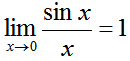注意，运动模糊后的图像的尺寸会变化，如果还是按照原图截取，会造成图像成分的损失，在复原图像时候效果不是太好，而且不知道导致效果不好的原因，是由于成分的缺失，还是噪声的干扰。所以，这里我适当的扩展了图像的尺寸，以保留图像的所有成分
此模型的执行结果如下所示。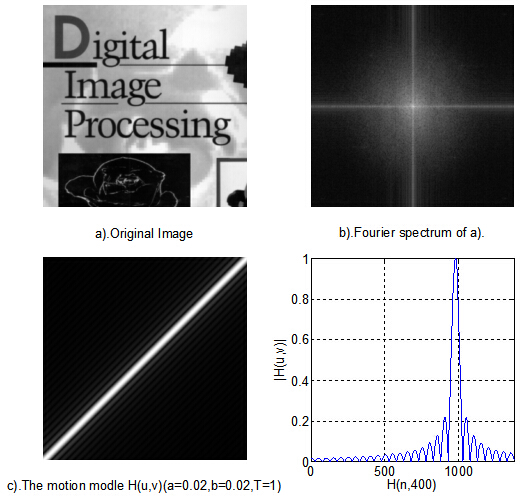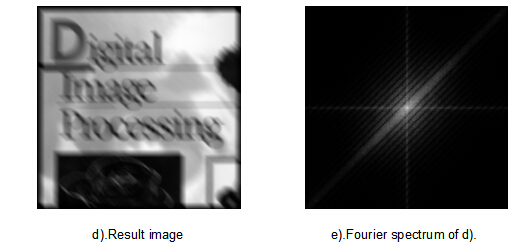3.图像的逆滤波

3.1 实验步骤与实验用图像

我们是这样的一个实验步骤，首先，使用退化函数处理图像，然后加上适当的可加性噪声。使用这样的图像进行逆滤波实验。
下面是实验用图像。图像的噪声选用的是高斯噪声，均值为0，方差为0.08。退化函数则选用先前叙述的两种，一个个大气湍流模型，一个是运动模糊。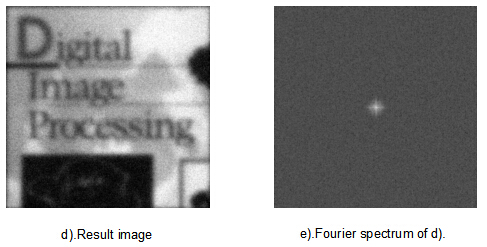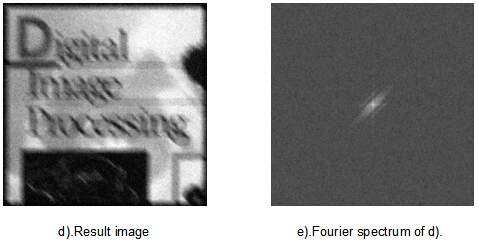3.2 直接逆滤波

所谓直接逆滤波，就是不管噪声的影响，直接进行逆滤波的方法。对于大气湍流模型而言，直接逆滤波会得到很不理想的结果。下面是直接逆滤波的实验结果。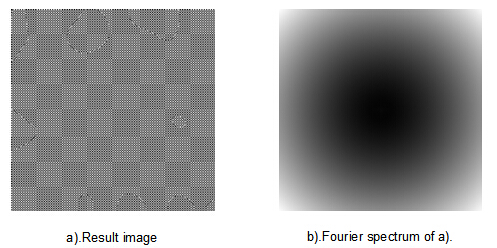实验结果完全没有任何价值。观察其频谱，频谱的四角很亮，而原本频谱最亮的直流分量都看不到了。所以，这里做一个限制处理。也就是，仅仅只处理靠近直流分量的部分，其他的不做处理。然后处理完的结果，过一个10阶巴特沃斯低通滤波器。可以得到如下结果。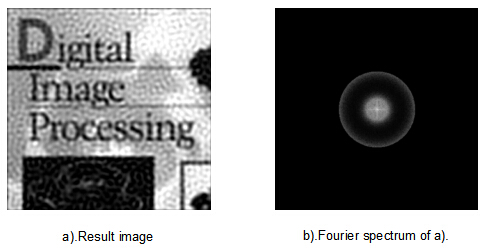这样的话，只需要调整限制半径，可以得到一个比之前较好的结果。当然，这招在运动模糊的图片面前，就略显得无力了。结果我就不贴了。

3.3 维纳滤波器

维纳滤波器的推导，其实是个很复杂的过程。这里就不推导了，直接看结果，可以得到一些有用的结论。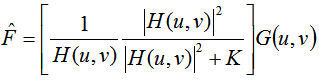观察式子，对于合适的常数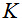，有如下两个结论。
1.对于退化函数很小的点，相对而言常数的值很大，其倒数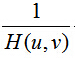不会太大。
2.对于退化函数很小的点，相对而言常数的值很小，其倒数基本保持不变。

下面的维纳滤波器的实验结果：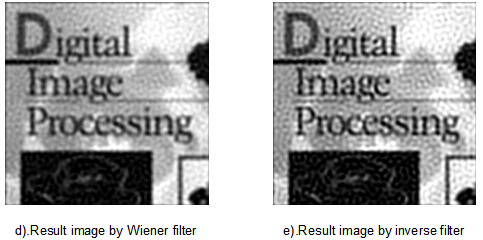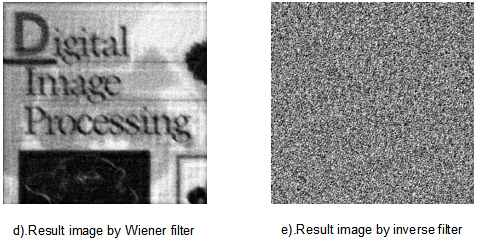3.4 约束最小二乘方滤波

其实这个方法是一个很好的想法，将图像的能量作为评价图像平滑程度的度量，尽可能的将其平滑。设噪声的能量是一个定值，使用拉普拉斯未定系数法，将其进行迭代，然后解开。 这种方法有了很多的变种，包括很著名的TV(Total Variation，全变分)模型，这个我之后的博文会讲到。
在这里，使用本方法的目的是，减少噪音对于逆滤波的影响。表达式如下所示。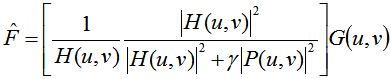这个滤波器，可以消除很严重的噪声，并且复原图像。将实验用图像的噪声的方差提升到0.2，再进行滤波，可以得到如下结果。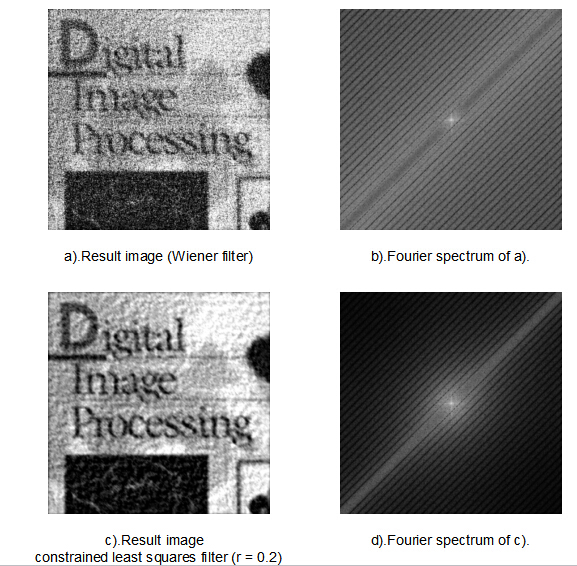4 实验代码

1. close all;
2. clear all;
3. clc;
4. %% ----------init-----------------------------
6. f = mat2gray(f,[ 0 255]);
7. f_original = f;
8. [M,N] = size(f);
9. P = 2*M;
10. Q = 2*N;
11. fc = zeros(M,N);
12. for x = 1: 1:M
13. for y = 1: 1:N
14. fc( x, y) = f( x, y) * (- 1)^( x+ y);
15. end
16. end
17. F_I = fft2(fc,P,Q);
18. figure();
19. subplot( 1, 2, 1);
20. imshow(f,[ 0 1]);
21. xlabel( 'a).Original Image');
22. subplot( 1, 2, 2);
23. imshow( log( 1 + abs(F_I)),[ ]);
24. xlabel( 'b).Fourier spectrum of a).');
25. %% ------motion blur------------------
26. H = zeros(P,Q);
27. a = 0. 02;
28. b = 0. 02;
29. T = 1;
30. for x = (-P/ 2): 1:(P/ 2)- 1
31. for y = (-Q/ 2): 1:(Q/ 2)- 1
32. R = ( x*a + y*b)*pi;
33. if(R == 0)
34. H( x+(P/ 2)+ 1, y+(Q/ 2)+ 1) = T;
35. else H( x+(P/ 2)+ 1, y+(Q/ 2)+ 1) = (T/R)*( sin(R))* exp(- 1i*R);
36. end
37. end
38. end
39. %% ------the atmospheric turbulence modle------------------
40. H_1 = zeros(P,Q);
41. k = 0. 0025;
42. for x = (-P/ 2): 1:(P/ 2)- 1
43. for y = (-Q/ 2): 1:(Q/ 2)- 1
44. D = ( x^ 2 + y^ 2)^( 5/ 6);
45. D_ 0 = 60;
46. H_1( x+(P/ 2)+ 1, y+(Q/ 2)+ 1) = exp(-k*D);
47. end
48. end
49. %% -----------noise------------------
50. a = 0;
51. b = 0. 2;
52. n_gaussian = a + b .* randn(M,N);
53. Noise = fft2(n_gaussian,P,Q);
54. figure();
55. subplot( 1, 2, 1);
56. imshow(n_gaussian,[- 1 1]);
57. xlabel( 'a).Gaussian noise');
58. subplot( 1, 2, 2);
59. imshow( log( 1 + abs(Noise)),[ ]);
60. xlabel( 'b).Fourier spectrum of a).');
61. %%
62. G = H .* F_I + Noise;
63. % G = H_1 .* F_I + Noise;
64. gc = ifft2(G);
65. gc = gc( 1: 1:M+ 27, 1: 1:N+ 27);
66. for x = 1: 1:(M+ 27)
67. for y = 1: 1:(N+ 27)
68. g( x, y) = gc( x, y) .* (- 1)^( x+ y);
69. end
70. end
71. gc = gc( 1: 1:M, 1: 1:N);
72. for x = 1: 1:(M)
73. for y = 1: 1:(N)
74. g( x, y) = gc( x, y) .* (- 1)^( x+ y);
75. end
76. end
77. figure();
78. subplot( 1, 2, 1);
79. imshow(f,[ 0 1]);
80. xlabel( 'a).Original Image');
81. subplot( 1, 2, 2);
82. imshow( log( 1 + abs(F_I)),[ ]);
83. xlabel( 'b).Fourier spectrum of a).');
84. figure();
85. subplot( 1, 2, 1);
86. imshow( abs(H),[ ]);
87. xlabel( 'c).The motion modle H(u,v)(a=0.02,b=0.02,T=1)');
88. subplot( 1, 2, 2);
89. n = 1: 1:P;
90. plot(n, abs(H( 400,:)));
91. axis([ 0 P 0 1]);grid;
92. xlabel( 'H(n,400)');
93. ylabel( '|H(u,v)|');
94. figure();
95. subplot( 1, 2, 1);
96. imshow(real(g),[ 0 1]);
97. xlabel( 'd).Result image');
98. subplot( 1, 2, 2);
99. imshow( log( 1 + abs(G)),[ ]);
100. xlabel( 'e).Fourier spectrum of d). ');
101. %% --------------inverse_filtering---------------------
102. %F = G ./ H;
103. %F = G ./ H_1;
104. for x = (-P/ 2): 1:(P/ 2)- 1
105. for y = (-Q/ 2): 1:(Q/ 2)- 1
106. D = ( x^ 2 + y^ 2)^( 0. 5);
107. if(D < 258)
108. F( x+(P/ 2)+ 1, y+(Q/ 2)+ 1) = G( x+(P/ 2)+ 1, y+(Q/ 2)+ 1) ./ H_1( x+(P/ 2)+ 1, y+(Q/ 2)+ 1);
109. % no noise D < 188
110. % noise D < 56
111. else F( x+(P/ 2)+ 1, y+(Q/ 2)+ 1) = G( x+(P/ 2)+ 1, y+(Q/ 2)+ 1);
112. end
113. end
114. end
115. % Butterworth_Lowpass_Filters
116. H_B = zeros(P,Q);
117. D_ 0 = 70;
118. for x = (-P/ 2): 1:(P/ 2)- 1
119. for y = (-Q/ 2): 1:(Q/ 2)- 1
120. D = ( x^ 2 + y^ 2)^( 0. 5);
121. %if(D < 200) H_B( x+(P/ 2)+ 1, y+(Q/ 2)+ 1) = 1/( 1+(D/D_ 0)^ 100);end
122. H_B( x+(P/ 2)+ 1, y+(Q/ 2)+ 1) = 1/( 1+(D/D_ 0)^ 20);
123. end
124. end
125. F = F .* H_B;
126. f = real(ifft2(F));
127. f = f( 1: 1:M, 1: 1:N);
128. for x = 1: 1:(M)
129. for y = 1: 1:(N)
130. f( x, y) = f( x, y) * (- 1)^( x+ y);
131. end
132. end
133. %% ------show Result------------------
134. figure();
135. subplot( 1, 2, 1);
136. imshow(f,[ 0 1]);
137. xlabel( 'a).Result image');
138. subplot( 1, 2, 2);
139. imshow( log( 1 + abs(F)),[ ]);
140. xlabel( 'b).Fourier spectrum of a).');
141. figure();
142. n = 1: 1:P;
143. plot(n, abs(F( 400,:)), 'r-',n, abs(F( 400,:)), 'b-');
144. axis([ 0 P 0 1000]);grid;
145. xlabel( 'Number of rows(400th column)');
146. ylabel( 'Fourier amplitude spectrum');
147. legend( 'F_{limit}(u,v)', 'F(u,v)');
148. figure();
149. n = 1: 1:P;
150. plot(n, abs(H( 400,:)), 'g-');
151. axis([ 0 P 0 1]);grid;
152. xlabel( 'H' '_{s}(n,400)');
153. ylabel( '|H' '_{s}(u,v)|');
154. %% ----------Wiener filters-----------
155. % K = 0. 000014;
156. K = 0. 02;
157. %H_Wiener = (( abs(H_1).^ 2)./(( abs(H_1).^ 2)+K)).*( 1./H_1);
158. H_Wiener = (( abs(H).^ 2)./(( abs(H).^ 2)+K)).*( 1./H);
159. F_Wiener = H_Wiener .* G;
160. f_Wiener = real(ifft2(F_Wiener));
161. f_Wiener = f_Wiener( 1: 1:M, 1: 1:N);
162. for x = 1: 1:(M)
163. for y = 1: 1:(N)
164. f_Wiener( x, y) = f_Wiener( x, y) * (- 1)^( x+ y);
165. end
166. end
167. [SSIM_Wiener mssim] = ssim_index(f_Wiener,f_original,[ 0. 01 0. 03],ones( 8), 1);
168. SSIM_Wiener
169. %% ------show Result------------------
170. figure();
171. subplot( 1, 2, 1);
172. %imshow(f_Wiener( 1: 128, 1: 128),[ 0 1]);
173. imshow(f_Wiener,[ 0 1]);
174. xlabel( 'd).Result image by Wiener filter');
175. subplot( 1, 2, 2);
176. imshow( log( 1+ abs(F_Wiener)),[ ]);
177. xlabel( 'c).Fourier spectrum of c).');
178. % subplot( 1, 2, 2);
179. % %imshow(f( 1: 128, 1: 128),[ 0 1]);
180. % imshow(f,[ 0 1]);
181. % xlabel( 'e).Result image by inverse filter');
182. figure();
183. n = 1: 1:P;
184. plot(n, abs(F( 400,:)), 'r-',n, abs(F_Wiener( 400,:)), 'b-');
185. axis([ 0 P 0 500]);grid;
186. xlabel( 'Number of rows(400th column)');
187. ylabel( 'Fourier amplitude spectrum');
188. legend( 'F(u,v)', 'F_{Wiener}(u,v)');
189. figure();
190. subplot( 1, 2, 1);
191. imshow( log( 1 + abs(H_Wiener)),[ ]);
192. xlabel( 'a).F_{Wiener}(u,v).');
193. subplot( 1, 2, 2);
194. n = 1: 1:P;
195. plot(n, abs(H_Wiener( 400,:)));
196. axis([ 0 P 0 80]);grid;
197. xlabel( 'Number of rows(400th column)');
198. ylabel( 'Amplitude spectrum');
199. %% ------------Constrained_least_squares_filtering---------
200. p_laplacian = zeros(M,N);
201. Laplacian = [ 0 - 1 0;
202. - 1 4 - 1;
203. 0 - 1 0];
204. p_laplacian( 1: 3, 1: 3) = Laplacian;
205. P = 2*M;
206. Q = 2*N;
207. for x = 1: 1:M
208. for y = 1: 1:N
209. p_laplacian( x, y) = p_laplacian( x, y) * (- 1)^( x+ y);
210. end
211. end
212. P_laplacian = fft2(p_laplacian,P,Q);
213. F_C = zeros(P,Q);
214. r = 0. 2;
215. H_clsf = ((H ')./((abs(H).^2)+r.*P_laplacian));
216. F_C = H_clsf .* G;
217. f_c = real(ifft2(F_C));
218. f_c = f_c(1:1:M,1:1:N);
219. for x = 1:1:(M)
220. for y = 1:1:(N)
221. f_c(x,y) = f_c(x,y) * (-1)^(x+y);
222. end
223. end
224. %%
225. figure();
226. subplot(1,2,1);
227. imshow(f_c,[0 1]);
228. xlabel('e).Result image by constrained least squares filter (r = 0. 2) ');
229. subplot(1,2,2);
230. imshow(log(1 + abs(F_C)),[ ]);
231. xlabel('f).Fourier spectrum of c). ');
232. [SSIM_CLSF mssim] = ssim_index(f_c,f_original,[0.01 0.03],ones(8),1);
233. figure();
234. subplot(1,2,1);
235. imshow(log(1 + abs(H_clsf)),[ ]);
236. xlabel('a).F _ {clsf}(u,v). ');
237. subplot(1,2,2);
238. n = 1:1:P;
239. plot(n,abs(H_clsf(400,:)));
240. axis([0 P 0 80]);grid;
241. xlabel('Number of rows( 400th column) ');
242. ylabel('Amplitude spectrum ');

=============更新日志===================

NULL

展开全文• 逆滤波和维纳滤波

千次阅读 2020-06-09 15:27:41
利用逆滤波和维纳滤波，对Lena加噪运动模糊降质图像进行复原，比较不同参数选择对复原结果的影响。二、实验内容1)     输入Lena图像，对图像进行运动降质；降质模型：2) ...

一、实验目的

利用逆滤波和维纳滤波，对Lena加噪运动模糊降质图像进行复原，比较不同参数选择对复原结果的影响。

二、实验内容

1)     输入Lena图像，对图像进行运动降质；降质模型：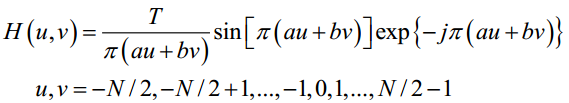2)     对图像叠加高斯白噪声；

3)     寻找最佳逆滤波半径r；

4)     逆滤波；

5)     IFFT，展示结果；

6)     再寻找最佳维纳滤波K值；

7)     维纳滤波；

8)     IFFT，展示结果。

三、实验过程和数学原理

1、逆滤波

在无噪情况下，逆滤波是完美的：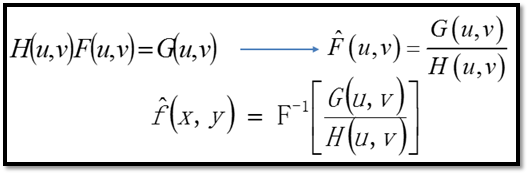然而实际情况都有噪声：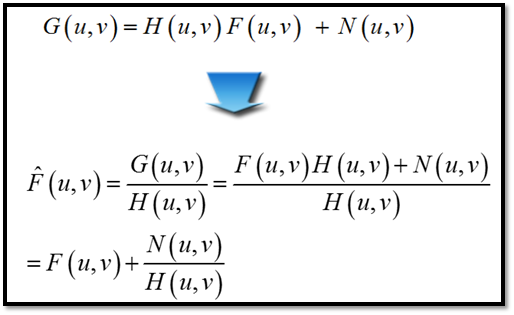如果H(u,v)存在零点，那么在H(u,v)零点附近进行复原，会导致第二项变得很大很大，复原效果很差。

理论上说，我们应该找出H(u,v)的所有零点，然后规避这些零点进行逆滤波。然而，降质模型零点十分分散（实际上是一条条斜线，实验中会看到），并且要作无数个圆区域，编程非常麻烦。

实际编程中，我们采用如下思路：既然fft后的频谱中，信号频谱主要集中在低频分量，那么，我们用fftshift，将频谱移到中心；以频谱中心为圆心，规定一个圆区域，在圆内正常逆滤波；而圆外是大量的较小的噪声分量，不给任何机会，直接赋值为0。这样，我们就不需要考虑H(u,v)的零点影响了。

此外，再通过PSNR最大准则，寻找最佳滤波半径R。实验结果如下：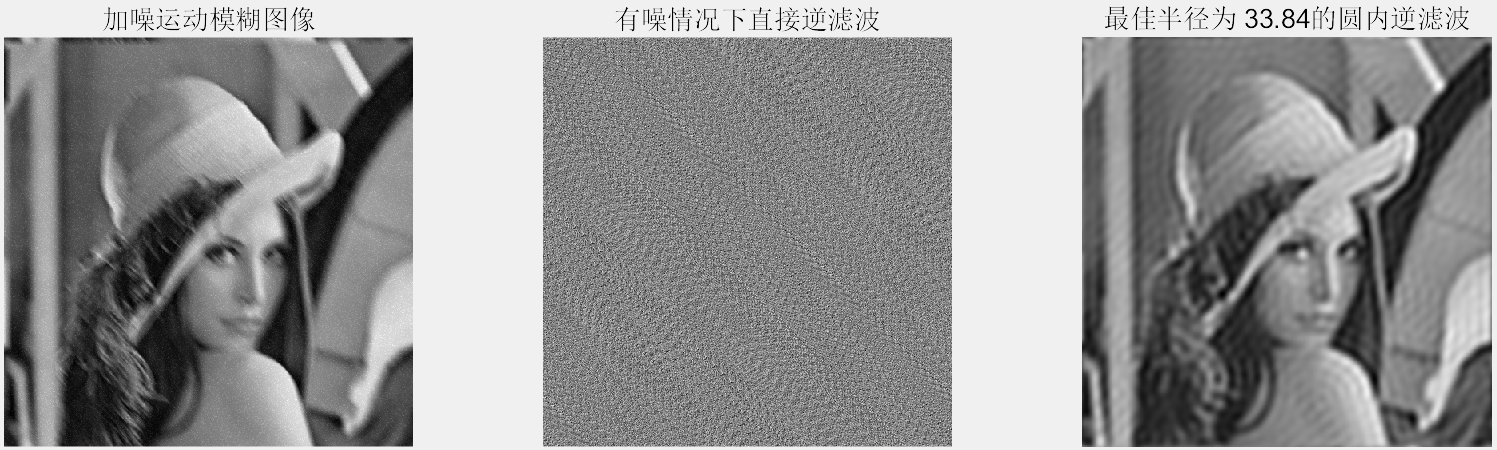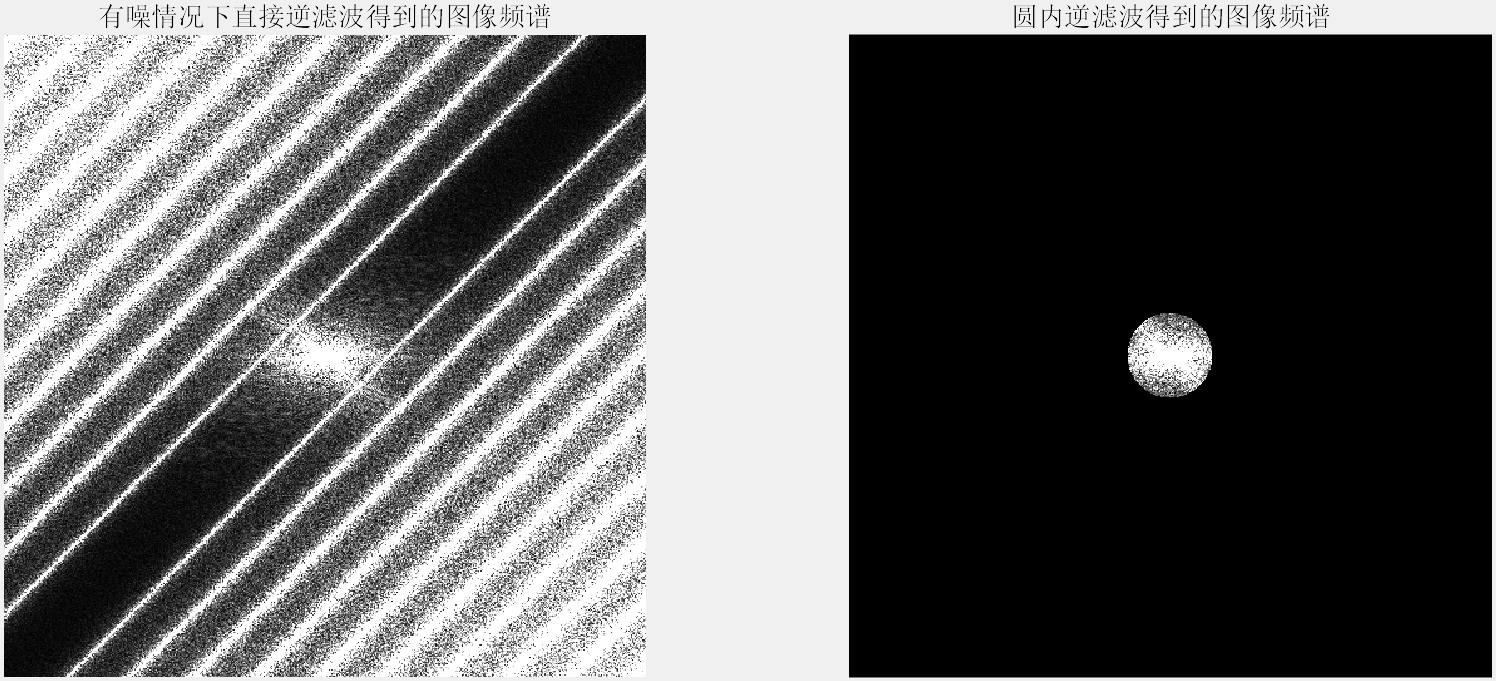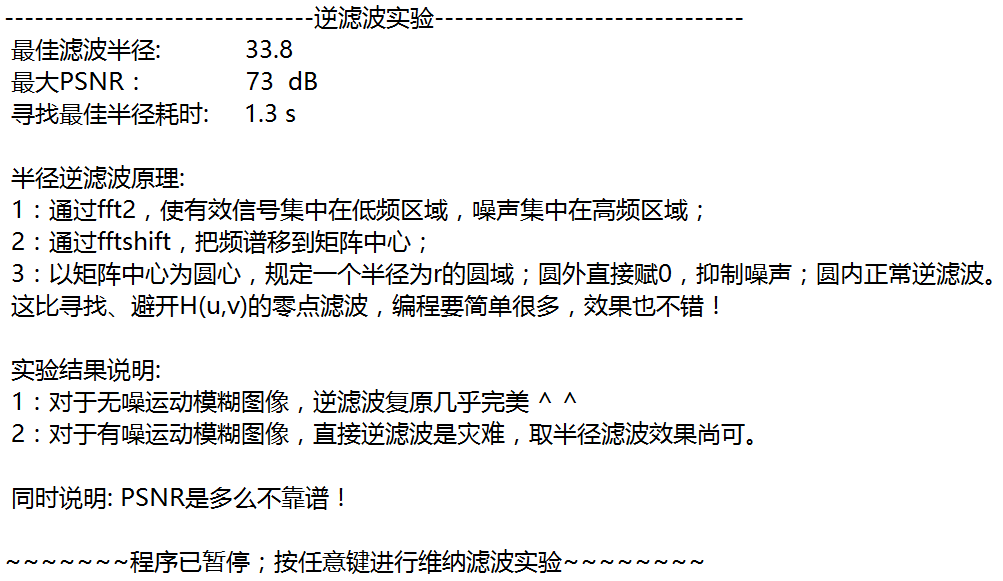实验代码：

function Imagerestoration
%~~~~~~~~~~~~~~~~~~~~~~~~~~实验说明~~~~~~~~~~~~~~~~~~~~~~~~~~~~~~~~~~%
% 1、由于T=5,a=b=1效果太差太差，几乎无法看到复原现象，因此本实验采用T=1,a=b=0.02降质模型。
% 2、作为实验讲义的补充，本实验加入均值为0、方差为1e-3的AGWN模型。
%       若方差过大，逆滤波效果也不理想。
% 3、一定要对fftshift后的频谱进行运动模糊处理！！！否则现象都是错误的！！！
%                                                                                                                                       2018-5-19 by XING
%~~~~~~~~~~~~~~~~~~~~~~~~~~~~~~~~~~~~~~~~~~~~~~~~~~~~~~~~~~~~~~~~~~%
clear;close all;clc;
fprintf('-------------------------------逆滤波实验-------------------------------\n');
[M,~] = size(I);% square

% Display the original image.
figure;
subplot(1,3,1), imshow(I);
title(’\fontsize{20}原始图像’);

%% Simulate a Motion Blur：H(u,v)
T=1;a=0.02;b=0.02;
v=[-M/2:M/2-1];u=v;
A=repmat(a.u,1,M)+repmat(b.v,M,1);
H=T/pi./A.sin(pi.A).exp(-1ipi.*A);
H(A==0)=T;% replace NAN

%% Get the blurred Image
% Warning: fftshift should be written
F=fftshift(fft2(I));
FBlurred=F.*H;

% Display the blurred image
IBlurred =real(ifft2(ifftshift(FBlurred)));
subplot(1,3,2), imshow(uint8(255.*mat2gray(IBlurred)));
title(’\fontsize{20}运动模糊图像’);

%% Deblur perfectly without Noise
FDeblurred=FBlurred./H;
IDeblurred=real(ifft2(ifftshift(FDeblurred)));
subplot(1,3,3), imshow(uint8(255.*mat2gray(IDeblurred)));
title(’\fontsize{20}无噪情况下直接逆滤波’);

%% Simulate Noise Model
noise_mean = 0;
noise_var = 1e-3;
noise=imnoise(zeros(M),‘gaussian’, noise_mean,noise_var);
FNoise=fftshift(fft2(noise));

%% Get the Blurred_Noised Image
FBlurred_Noised=FNoise+FBlurred;

% Display the blurred_noised image
IBlurred_Noised=real(ifft2(ifftshift(FBlurred_Noised)));
figure;
subplot(1,3,1), imshow(uint8(255.*mat2gray(IBlurred_Noised)));
title(’\fontsize{20}加噪运动模糊图像’);

%% Deblur when Ignoring Noise
FDeblurred2=FBlurred_Noised./H;
FH1=abs(FDeblurred2);
IDeblurred2=real(ifft2(ifftshift(FDeblurred2)));
subplot(1,3,2), imshow(uint8(255.*mat2gray(IDeblurred2)));
title (’\fontsize{20}有噪情况下直接逆滤波’);

%% Find out the best Radius
maxPSNR=0;
tic;
FDeblurred2=zeros(M);

<span class="k" style="color:rgb(0,112,32);font-weight:bold;">for</span> <span class="n">a</span><span class="p">=</span><span class="mi" style="color:rgb(64,160,112);">1</span><span class="p">:</span><span class="n">M</span>
<span class="k" style="color:rgb(0,112,32);font-weight:bold;">for</span> <span class="n">b</span><span class="p">=</span><span class="mi" style="color:rgb(64,160,112);">1</span><span class="p">:</span><span class="n">M</span>
<span class="k" style="color:rgb(0,112,32);font-weight:bold;">if</span> <span class="nb" style="color:rgb(0,112,32);">sqrt</span><span class="p">((</span><span class="n">a</span><span class="o" style="color:rgb(102,102,102);">-</span><span class="n">M</span><span class="o" style="color:rgb(102,102,102);">/</span><span class="mi" style="color:rgb(64,160,112);">2</span><span class="p">)</span><span class="o" style="color:rgb(102,102,102);">.^</span><span class="mi" style="color:rgb(64,160,112);">2</span><span class="o" style="color:rgb(102,102,102);">+</span><span class="p">(</span><span class="n">b</span><span class="o" style="color:rgb(102,102,102);">-</span><span class="n">M</span><span class="o" style="color:rgb(102,102,102);">/</span><span class="mi" style="color:rgb(64,160,112);">2</span><span class="p">)</span><span class="o" style="color:rgb(102,102,102);">.^</span><span class="mi" style="color:rgb(64,160,112);">2</span><span class="p">)</span><span class="o" style="color:rgb(102,102,102);">&lt;</span><span class="n">Radius</span>
<span class="n">FDeblurred2</span><span class="p">(</span><span class="n">a</span><span class="p">,</span><span class="n">b</span><span class="p">)=</span><span class="n">FBlurred_Noised</span><span class="p">(</span><span class="n">a</span><span class="p">,</span><span class="n">b</span><span class="p">)</span><span class="o" style="color:rgb(102,102,102);">./</span><span class="n">H</span><span class="p">(</span><span class="n">a</span><span class="p">,</span><span class="n">b</span><span class="p">);</span>
<span class="k" style="color:rgb(0,112,32);font-weight:bold;">end</span>
<span class="k" style="color:rgb(0,112,32);font-weight:bold;">end</span>
<span class="k" style="color:rgb(0,112,32);font-weight:bold;">end</span>

<span class="c" style="color:rgb(96,160,176);font-style:italic;">% Calculate PSNR and compare with the best</span>
<span class="n">IDeblurred2</span><span class="p">=</span><span class="nb" style="color:rgb(0,112,32);">real</span><span class="p">(</span><span class="n">ifft2</span><span class="p">(</span><span class="n">ifftshift</span><span class="p">(</span><span class="n">FDeblurred2</span><span class="p">)));</span>
<span class="n">PSNR</span><span class="p">=</span><span class="n">PSNRcal</span><span class="p">(</span><span class="n">IDeblurred2</span><span class="p">,</span><span class="n">I</span><span class="p">);</span>
<span class="k" style="color:rgb(0,112,32);font-weight:bold;">if</span> <span class="n">PSNR</span><span class="o" style="color:rgb(102,102,102);">&gt;</span><span class="n">maxPSNR</span>
<span class="n">maxPSNR</span><span class="p">=</span><span class="n">PSNR</span><span class="p">;</span>
<span class="k" style="color:rgb(0,112,32);font-weight:bold;">end</span>

end

fprintf(’ 最大PSNR： %d dB\n’, round(maxPSNR));
fprintf(’ 寻找最佳半径耗时: %.1f s\n’, toc);

% Displace the best Restoration
FDeblurred2=zeros(M);
for a=1:M
for b=1:M
FDeblurred2(a,b)= FBlurred_Noised(a,b)./H(a,b);
end
end
end

FH2=abs(FDeblurred2);

IDeblurred2=real(ifft2(ifftshift(FDeblurred2)));
subplot(1,3,3), imshow(uint8(255.*mat2gray(IDeblurred2)));

fprintf(’\n 半径逆滤波原理: \n’);
fprintf(’ 1：通过fft2，使有效信号集中在低频区域，噪声集中在高频区域；\n’);
fprintf(’ 2：通过fftshift，把频谱移到矩阵中心；\n’);
fprintf(’ 3：以矩阵中心为圆心，规定一个半径为r的圆域；圆外直接赋0，抑制噪声；圆内正常逆滤波。\n’);
fprintf(’ 这比寻找、避开H(u,v)的零点滤波，编程要简单很多，效果也不错！\n’);

fprintf(’\n 实验结果说明: \n’);
fprintf(’ 1：对于无噪运动模糊图像，逆滤波复原几乎完美 ^ ^\n’);
fprintf(’ 2：对于有噪运动模糊图像，直接逆滤波是灾难，取半径滤波效果尚可。\n’);
fprintf(’\n 同时说明: PSNR是多么不靠谱！\n’);

figure;
subplot(1,2,1),imshow(im2double(uint8(FH1)));
title (’\fontsize{20}有噪情况下直接逆滤波得到的图像频谱’);
subplot(1,2,2),imshow(im2double(uint8(FH2)));
title (’\fontsize{20}圆内逆滤波得到的图像频谱’);

fprintf(’\n~程序已暂停；按任意键进行维纳滤波实验~~\n’);
pause;

2、维纳滤波

理想维纳滤波器为：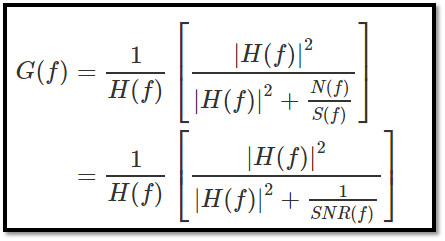实际应用中，NSR难以被精确计算。因此，我们常常设为K，并寻找最佳K值。

实验结果：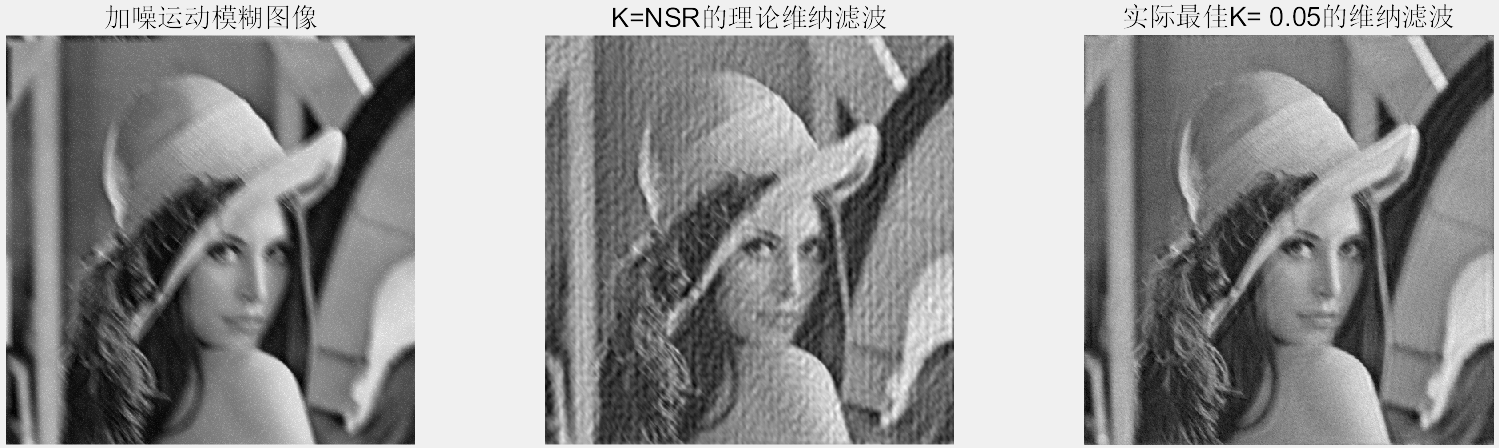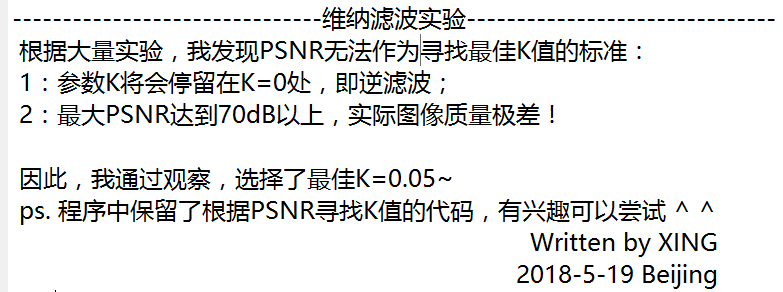实验代码：

%% Deblur Image Using Wiener Filter
fprintf(’\n-------------------------------维纳滤波实验-------------------------------\n’);
fprintf(’ 根据大量实验，我发现PSNR无法作为寻找最佳K值的标准：\n’);
fprintf(’ 1：参数K将会停留在K=0处，即逆滤波；\n’);
fprintf(’ 2：最大PSNR达到70dB以上，实际图像质量极差！\n’);
fprintf(’\n 因此，我通过观察，选择了最佳K=0.05~\n’);
fprintf(’ ps. 程序中保留了根据PSNR寻找K值的代码，有兴趣可以尝试 ^ ^\n’);
% Display the blurred_noised image again
figure();
subplot(1,3,1);
imshow(uint8(255.*mat2gray(IBlurred_Noised)));
title(’\fontsize{20}加噪运动模糊图像’);

% Deblur with theoretic NSR
buf=(abs(H)).^2; % Notice ‘.’ !!!
NSR=FNoise./F;
FDeblurred3=FBlurred_Noised./H.buf./(buf+NSR);
IDeblurred3=real(ifft2(ifftshift(FDeblurred3)));
subplot(1,3,2), imshow(uint8(255.mat2gray(IDeblurred3)));
title(’\fontsize{20}K=NSR的理论维纳滤波’);

% Find out the best K
% tic;
% maxPSNR=0;
% beskK=0;
% for K=0:1e-2:1
% FDeblurred2=zeros(M);
% FDeblurred3=FBlurred_Noised./H.*buf./(buf+bestK);
% IDeblurred3=real(ifft2(ifftshift(FDeblurred3)));
%
% % Calculate PSNR and compare with the best
% PSNR=PSNRcal(IDeblurred3,I);
% if PSNR>maxPSNR
% maxPSNR=PSNR;
% bestK=K;
% end
% end
%
% fprintf(’ 最佳K值: %.2f\n’, bestK);
% fprintf(’ 最大PSNR: %d dB\n’, round(maxPSNR));
% fprintf(’ 寻找最佳K值耗时: %.1f s\n’, toc);

% Deblur with best K
bestK=0.05;
FDeblurred3=FBlurred_Noised./H.*buf./(buf+bestK);
IDeblurred3=real(ifft2(ifftshift(FDeblurred3)));

% Display the best restored Image
subplot(1,3,3), imshow(uint8(255.*mat2gray(IDeblurred3)));
title([’\fontsize{20}实际最佳K= ', num2str(bestK),‘的维纳滤波’]);

fprintf(’ Written by XING\n’);
fprintf(’ 2018-5-19 Beijing\n’);
end

实验中用到的PSNR计算子程序：

function PSNR=PSNRcal(I,I2)
h=512;w=512;
B=8;% 编码一个像素用8个二进制位
MAX=2^B-1;% 图像有多少灰度级
MES=sum(sum((I-I2).^2))/(hw);% 均方差
PSNR=20log10(MAX/sqrt(MES));% 峰值信噪比
end

展开全文• 【图像修复】图像运动模糊消除(逆滤波)matlab源码.md
• 逆滤波和维纳滤波 简介 在图像的获取、传输以及记录保存过程中，由于各种因素，如成像设备与目标物体的相对运动，大气的湍流效应，光学系统的相差，成像系统的非线性畸变，环境的随机噪声等原因都会使图像产生一定...

逆滤波和维纳滤波

简介

在图像的获取、传输以及记录保存过程中，由于各种因素，如成像设备与目标物体的相对运动，大气的湍流效应，光学系统的相差，成像系统的非线性畸变，环境的随机噪声等原因都会使图像产生一定程度的退化，图像退化的典型表现是图像出现模糊、失真，出现附加噪声等。由于图像的退化，使得最终获取的图像不再是原始图像，图像效果明显变差。为此， 要较好地显示原始图像，必须对退化后的图像进行处理，恢复出真实的原始图像，这一过程就称为图像复原。

大气湍流退化

对经过大气湍流退化的图片实现全逆滤波，半径受限逆滤波以及维纳滤波，并对比。

三种滤波思想

直接全逆滤波、半径受限逆滤波和维纳滤波

直接全逆滤波

如果退化图像为 g(x, y)，原始图像为 f (x, y)，在不考虑噪声的情况下，由傅立叶变换的卷积定理可知有下式成立 G(u, v) = H(u, v) ∗ F (u, v)。由此可见，如果已知退化图像的傅里叶变换和系统的冲击响应函数 G(u, v)，则可以求出原图像的傅里叶变换 H(u, v)，之后使用傅里叶逆变换即可得到原图像。这就是全逆滤波的主要思想。

半径受限逆滤波

全逆滤波在有噪声的情况下：
F ( u , v ) = G ( u , v ) / H ( u , v ) F (u, v) = G(u, v) /H(u, v)
F ’ ( u , v ) = G ( u , v ) / H ( u , v ) − N ( u , v ) / H ( u , v ) F ’(u, v) =G(u, v)/H(u, v) −N (u, v) /H(u, v)
N (u, v) 是噪声的傅里叶变换。如果此时在 N (u, v) 不为 0 的地方，H(u, v) 过零或者比较小， 则会导致噪声被放大。因此对 F (u, v) 首先使用低通滤波器过滤掉高频的噪声，之后再除以H(u, v)，这样虽然有可能会导致图像的高频细节被忽略，但是相对于全逆滤波更加稳定。

维纳滤波

维纳滤波就是最小二乘滤波，它是使原始图像 f(x,y) 与其恢复图像 f’(x,y) 之间的均方误差最小的复原方法。具有较好的抑制噪音的能力。由于在日常使用中，无法确定图片的信噪比，因此在公式中使用参数 k 来代替信噪比。其公式为：
F ’ ( u , v ) = 1 H ( u , v ) ∗ ∣ H ( u , v ) ∣ 2 ∣ H ( u , v ) ∣ 2 + k F’(u,v) = \frac{1}{H(u,v)} * \frac{|H(u,v)|^{2}}{|H(u,v)|^{2} + k}

代码1

主函数

clc;
clear;
close all;

%% 课本图 5.28
% 读取图片
im = imread('demo-1.jpg');   % 原始图像 480x480 uint8

%% 图像退化（大气湍流模型）
% Output（H：退化模型， im_f：退化后图片）
[H, im_f,im_F] = atmosph(im);

%% 全逆滤波，半径受限逆滤波
D0 = 60;
% Input（im_f：退化图片，H：退化模型，D0：半径）
% Output（im_inverse：全逆滤波结果，im_inverse_b：半径受限逆滤波）
[im_inverse, im_inverse_b] = my_inverse(im_f, H, D0);
% [im_inverse, im_inverse_b] = my_inverse_F(im_f, H, D0,0.001);

%% 维纳滤波
K = 0.0001;
% Input（im_f：退化图片，H：退化模型，K：维纳滤波常数）
im_wiener = my_wiener(im_f, H, K);

%% 显示结果
figure(1);
subplot(231); imshow(im); title('原图'); axis on
subplot(232); imshow(im_f); title('大气湍流(k=0.0025)'); axis on
subplot(233); imshow(im_inverse); title('全逆滤波'); axis on
subplot(234); imshow(im_inverse_b); title('半径受限的逆滤波'); axis on
subplot(235); imshow(im_wiener); title('维纳滤波'); axis on

全逆滤波和半径受限的全逆滤波

function [im_inverse, im_inverse_b] = my_inverse(img, H, D0)

[M,N] = size(img);

%图像进行二位傅里叶变换，之后移动到中心点
im_double = mat2gray(img,[0 255]);
im_F = fftshift(fft2(im_double));      % 空域 > 频域

% 10阶巴特沃斯低通滤波器
H2 = zeros(M,N);
for x = (-M/2):1:(M/2)-1
for y = (-N/2):1:(N/2)-1
D2 = (x^2 + y^2)^(1/2);
H2(x+(M/2)+1,y+(N/2)+1) = 1/(1+(D2/D0)^20);%十阶
end
end
%滤波后图像
im_H2 = im_F .* H2;

% 全逆滤波
% 抑制低幅值的频率的全逆滤波
% im_inverse = zeros(M,N);
% num_F = abs(im_F);
% for x = 1:M
%      for y = 1:N
%          if(num_F(x,y)>0.78)
%              im_inverse(x,y) = im_F(x,y) / H(x,y);
%          else
%              im_inverse(x,y) = im_F(x,y);
%          end
%      end
% end

%直接全逆滤波
im_inverse = im_F ./ H;

im_inverse_double = real(ifft2(ifftshift(im_inverse)));    % 频域 > 空域
im_inverse = im2uint8(mat2gray(im_inverse_double));

% 半径受限逆滤波
% 抑制低幅值的频率的全逆滤波
% im_inverse_b = zeros(M,N);
% num_H2 = abs(im_H2);
% for x = 1:M
%      for y = 1:N
%          if(num_H2(x,y)>0.78)
%              im_inverse_b(x,y) = im_H2(x,y) / H(x,y);
%          else
%              im_inverse_b(x,y) = im_H2(x,y);
%          end
%      end
% end
% 直接全逆滤波
im_inverse_b = im_H2 ./ H;
im_inverse_b_double = real(ifft2(ifftshift(im_inverse_b)));    % 频域 > 空域
im_inverse_b = im2uint8(mat2gray(im_inverse_b_double));
end

实验结果1

在本次实验中，首先对原图进行大气湍流退化（退化系数 k = 0.0025），之后分别对退化的图像进行全逆滤波，半径受限逆滤波（取半径 D0 = 60），维纳滤波（取信噪比 k = 0.0001）。

实验结果如下：如上图所示，实验中全逆滤波的结果是一团噪声，但是在图像退化过程中未引入噪声。在 实验中，经过反复调试，终于发现问题出在图像退化时的傅里叶变换的时候。在图像退化的反傅里叶变换时，存在一次对图像的取实部，这个操作没有问题，但是由于图像是离散的，当图像再次傅里叶变换，会在各个频段出现看似随机的噪声。这直接导致了由于 H(u,v) 过零导致的噪声放大。
为了说明全逆滤波对无噪声图像有着极好的恢复能力，重写给定退化函数，增加返还量im_F，不做反变换，用来使用全逆滤波。新的实验结果如下图：可以看出此时全逆滤波的效果很明显。

实验分析1

从上述实验结果可以看出，全逆滤波对无噪声的图像，具有极好的恢复能力，但是及其不稳定，可能由于各种各样的噪声，导致图像不仅没有变得清晰，反而变的更加模糊。而半径受限的全逆滤波，尽管理想的效果不如全逆滤波，会丢失很多细节，但是对于噪声的忍耐能力更强，相对也更加稳定。从上面比较来看，维纳滤波是效果最好的，并且其对噪声的抑制能力最强，但是需要知道图像的信噪比，才能最好的滤波。

运动模糊和高斯噪声污染

运动模糊是图像中的对象由于平移运动，导致的多幅图像的线性堆积。高斯噪声是服从高斯分布的亮度随机变化，是图像中常见的噪声污染。

运动模糊和高斯噪声设计思路

运动模糊是由于物体运动导致的，高斯噪声是一种由于设备、环境导致的随机性误差。

代码2

主函数

clc;
clear;
close all;

%% 课本图 5.29
% 读取图片
im = imread('demo-2.jpg');   % 原始图像 688x688 uint8

%% 图像退化（运动模糊+高斯噪声）
% [~, im1_f] = motionblur(im, 0.01);        % 噪声方差=0.01
% [~, im2_f] = motionblur(im, 0.001);
% [H, im3_f] = motionblur(im, 0.0000001);        % H：退化模型
[~, im1_f,im1_F] = motionblur(im, 0.01);        % 噪声方差=0.01
[~, im2_f,im2_F] = motionblur(im, 0.001);
[H, im3_f,im3_F] = motionblur(im, 0.0000001);        % H：退化模型

%% 全逆滤波，半径受限逆滤波
D0 = 33;
[~, im1_inverse] = my_inverse(im1_f, H, D0);
[~, im2_inverse] = my_inverse(im2_f, H, D0);
[~, im3_inverse] = my_inverse(im3_f, H, D0);
%  [im1_inverse, ~] = my_inverse(im1_f, H, D0);
%  [im2_inverse, ~] = my_inverse(im2_f, H, D0);
%  [im3_inverse, ~] = my_inverse(im3_f, H, D0);
%  [~, im1_inverse] = my_inverse_F(im1_f, H, 33);
%  [~, im2_inverse] = my_inverse_F(im2_f, H, 33);
%  [~, im3_inverse] = my_inverse_F(im3_f, H, 33);
%  [im1_inverse, ~] = my_inverse_F(im1_f, H, D0 ,0.95);
%  [im2_inverse, ~] = my_inverse_F(im2_f, H, D0 ,0.31);
%  [im3_inverse, ~] = my_inverse_F(im3_f, H, D0 ,0.005);

%% 维纳滤波
%K = 0.0001;%0.1 0.008 0.0001
im1_wiener = my_wiener(im1_f, H, 0.1);
im2_wiener = my_wiener(im2_f, H, 0.008);
im3_wiener = my_wiener(im3_f, H, 0.00001);

%% 显示结果
figure(1);
subplot(331); imshow(im1_f); title('运动模糊+加性噪声(sigma)'); axis on
subplot(332); imshow(im1_inverse); title('逆滤波结果'); axis on
subplot(333); imshow(im1_wiener); title('维纳滤波结果'); axis on
subplot(334); imshow(im2_f); title('运动模糊+加性噪声(sigma*0.1)'); axis on
subplot(335); imshow(im2_inverse); title('逆滤波结果'); axis on
subplot(336); imshow(im2_wiener); title('维纳滤波结果'); axis on
subplot(337); imshow(im3_f); title('运动模糊+加性噪声(sigma*0.00001)'); axis on
subplot(338); imshow(im3_inverse); title('逆滤波结果'); axis on
subplot(339); imshow(im3_wiener); title('维纳滤波结果'); axis on

运动模糊

function [H,im_blured, im_blured_F] = motionblur(img, sigma)

[M,N] = size(img);
%图像进行二位傅里叶变换，之后移动到中心点
im_double = mat2gray(img,[0 255]);
im_F = fftshift(fft2(im_double));      % 空域 > 频域

H = zeros(M,N);
gauss = 0 + sqrt(sigma) * randn(M,N);%二维高斯分布矩阵 0是均值
% 运动模糊
a = 0.1;
b = 0.1;
T = 1;

for u = -M/2:1:M/2-1
for v = -N/2:1:N/2-1
L = ((a*u)+(b*v))*pi;
if (L == 0)
H(u + M/2 + 1,v + N/2 + 1) = 1;
else
H(u + M/2 + 1,v + N/2 + 1) = T * sin(L) * exp( -L * 1i )/L;
end
end
end

im_G = im_F .* H;
%im_double = real(ifft2(ifftshift(im_G)));    % 频域 > 空域

% noise
im_gauss = fftshift(fft2(gauss));      % 空域 > 频域

im_blured_F = im_G + im_gauss;
%im_blured_F = im_G;
im_blured = real(ifft2(ifftshift(im_blured_F)));    % 频域 > 空域
end

维纳滤波

function im_wiener = my_wiener(img, H, K)
[M,N] = size(img);
H2 = zeros(M,N);
Mo = zeros(M,N);
Me = zeros(M,N);
% 空域 > 频域
im_double = mat2gray(img,[0 255]);
im_G = fftshift(fft2(im_double));

%得到系数H2
for u = 1 : M
for v = 1 : N
Mo(u,v) = (abs(H(u,v)))^2;
Me(u,v) = (H(u,v)*(Mo(u,v) + K));
H2(u,v) = Mo(u,v)/Me(u,v);
end
end

im_F = im_G.* H2;
im_wiener_double = real(ifft2(ifftshift(im_F)));    % 频域 > 空域
im_wiener = im2uint8(mat2gray(im_wiener_double));
end

实验结果2

首先是按照实验模板做了第一次实验，在实验中给三种情况下的维纳滤波分别调整的不同的信噪比系数 k，分别为 0.1、0.008、0.00001。但是针对逆滤波，无论是半径受限，还是未使用半径受限，都是一团噪声，无法产生需要的结果。如下图所示，（图 1 是全逆滤波，图 2是半径受限滤波）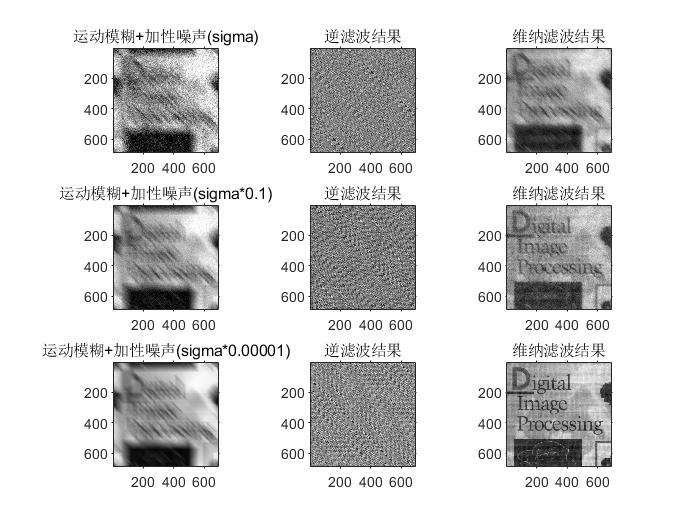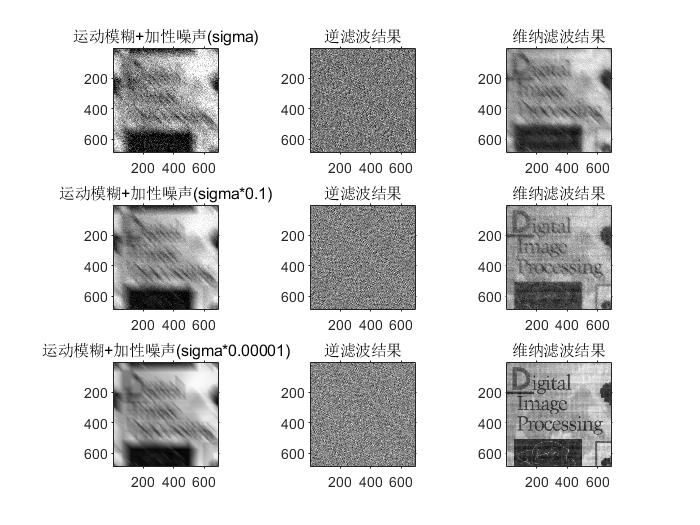实验思考

如上面所示，全滤波存在问题，但是按照理论知识，在噪声很小的情况下，逆滤波应该表现的相对清晰，因此，采用和大气湍流退化相同的处理方法，即对退化后的图像不进行反变换，直接全逆滤波处理，最终在半径受限的时候，得到了理想的处理结果，如下图所示：此时，笔者在反复分析图像处理过程时，发现对于这种程度的高斯噪声，其影响的频率点的幅值不会特别高。因此，笔者写了一个低幅值抑制的函数先对图像进行处理，然后对图像使用逆滤波，最后得到了细节保留优于维纳滤波的处理算法。下面是处理的函数和算法。本次实验的下限 k 分别是0.95，0.31，0.005。

function [im_inverse, im_inverse_b] = my_inverse_F(img, H, D0, k)

[M,N] = size(img);
%图像进行二位傅里叶变换，之后移动到中心点
im_double = mat2gray(img,[0 255]);
im_F = fftshift(fft2(im_double));      % 空域 > 频域
% im_F = img;
% 10阶巴特沃斯低通滤波器
H2 = zeros(M,N);
for x = (-M/2):1:(M/2)-1
for y = (-N/2):1:(N/2)-1
D2 = (x^2 + y^2)^(1/2);
H2(x+(M/2)+1,y+(N/2)+1) = 1/(1+(D2/D0)^20);%十阶
end
end
%滤波后图像
im_H2 = im_F .* H2;

% 全逆滤波
% 抑制低幅值的频率的全逆滤波
im_inverse = zeros(M,N);
num_F = abs(im_F);
for x = 1:M
for y = 1:N
if(num_F(x,y)>k)
im_inverse(x,y) = im_F(x,y) / H(x,y);
else
im_inverse(x,y) = im_F(x,y);
end
end
end

%直接全逆滤波
%im_inverse = im_F ./ H;

im_inverse_double = real(ifft2(ifftshift(im_inverse)));    % 频域 > 空域
im_inverse = im2uint8(mat2gray(im_inverse_double));

% 半径受限逆滤波
% 抑制低幅值的频率的全逆滤波
im_inverse_b = zeros(M,N);
num_H2 = abs(im_H2);
for x = 1:M
for y = 1:N
if(num_H2(x,y)>k)
im_inverse_b(x,y) = im_H2(x,y) / H(x,y);
else
im_inverse_b(x,y) = im_H2(x,y);
end
end
end

% 直接全逆滤波
% im_inverse_b = im_H2 ./ H;

im_inverse_b_double = real(ifft2(ifftshift(im_inverse_b)));    % 频域 > 空域
im_inverse_b = im2uint8(mat2gray(im_inverse_b_double));
end

i f ( n u m F ( x , y ) > k ) 如 果 幅 值 大 于 下 限 k if (num_F (x, y) > k) 如果幅值大于下限 k i n v e r s e ( x , y ) = i m F ( x , y ) / H ( x , y ) ; 正 常 使 用 逆 滤 波 inverse(x, y) = im_F (x, y)/H(x, y);正常使用逆滤波 e l s e 否 则 ， 说 明 其 信 息 重 要 度 低 else 否则，说明其信息重要度低 i n v e r s e ( x , y ) = i m F ( x , y ) ; 保 持 原 值 inverse(x, y) = im_F (x, y); 保持原值实验分析2

这次实验发现，使用维纳滤波交互性的选择信噪比 K，可以得到较好的图像显示效果。如期望的，全逆滤波产生的图像质量非常差，即使使用了半径限制的逆滤波依旧会产生较大的噪声干扰。这是因为 H 的小值较多，会使噪声放大。

在实验中，通过限制低幅度值的频谱值，可以得到一定程度上优于维纳滤波的限制性逆滤波算法。但是这些滤波算法都没办法完全克服噪声的污染，随着加性的高斯噪声越来越大， 结果也越来越差。

实验小结

本次实验，研究了全逆滤波，半径受限的逆滤波，维纳滤波，以及最后通过图像的频谱图 设计出的频域幅度限制逆滤波算法。全逆滤波的抗干扰能力很差，但是如果是完全没噪声的图像，可以给出最好的修正效果。半径受限的逆滤波会损失高频的细节，但是可以有更好的抑制干扰的能力。维纳滤波要明显优于逆滤波，其通过修正信噪比 k 可以给出图像极好的恢复结果。

最后，通过图像的频谱图设计出的频域幅度限制逆滤波算法，可以通过对频谱幅值小的噪声有很好的过滤效果，尽管也会损失细节，但是可以通过设置噪声的下限来进行改善。在一定程度上，获得了比维纳滤波更好的结果。更换图片，重试发现算法有一定的普适性，具有不错的鲁棒性。

展开全文算法 计算机视觉 matlab
• 《用逆滤波和维纳滤波进行图像复原[稻谷书苑]》由会员分享，可在线阅读，更多相关《用逆滤波和维纳滤波进行图像复原[稻谷书苑](6页珍藏版)》请在人人文库网上搜索。1、用逆滤波和维纳滤波进行图像复原在图像的获取、...

《用逆滤波和维纳滤波进行图像复原[稻谷书苑]》由会员分享，可在线阅读，更多相关《用逆滤波和维纳滤波进行图像复原[稻谷书苑](6页珍藏版)》请在人人文库网上搜索。

1、用逆滤波和维纳滤波进行图像复原在图像的获取、传输以及记录保存过程中，由于各种因素，如成像设备与目标物体的相对运动，大气的湍流效应，光学系统的相差，成像系统的非线性畸变，环境的随机噪声等原因都会使图像产生一定程度的退化，图像退化的典型表现是图像出现模糊、失真，出现附加噪声等。由于图像的退化，使得最终获取的图像不再是原始图像，图像效果明显变差。为此，要较好地显示原始图像，必须对退化后的图像进行处理，恢复出真实的原始图像，这一过程就称为图像复原。图像复原技术是图像处理领域一类非常重要的处理技术，主要目的就是消除或减轻在图像获取及传输过程中造成的图像质量下降即退化现象，恢复图像的本来面目。图像复原的过。

2、程是首先利用退化现象的某种先验知识，建立退化现象的数学模型，然后再根据退化模型进行反向的推演运算，以恢复原来的景物图像。一、 实验目的1了解图像复原模型2了解逆滤波复原和维纳滤波复原3掌握维纳滤波复原、逆滤波的Matlab实现二、实验原理1、逆滤波复原如果退化图像为，原始图像为，在不考虑噪声的情况下，其退化模型可用下式表示(12-25)由傅立叶变换的卷积定理可知有下式成立(12-26)式中，、分别是退化图像、点扩散函数、原始图像的傅立叶变换。所以(12-27)由此可见，如果已知退化图像的傅立叶变换和系统冲激响应函数(“滤被”传递函数)，则可以求得原图像的傅立叶变换，经傅立叶反变换就可以求得原始。

3、图像，其中除以起到了反向滤波的作用。这就是逆滤波复原的基本原理。在有噪声的情况下，逆滤波原理可写成如下形式(12-28)式中，是噪声的傅立叶变换。2、维纳滤波复原维纳滤波就是最小二乘滤波，它是使原始图像与其恢复图像之间的均方误差最小的复原方法。对图像进行维纳滤波主要是为了消除图像中存在的噪声，对于线性空间不变系统，获得的信号为(12-29)为了去掉中的噪声，设计一个滤波器，其滤波器输出为，即 (12-30)使得均方误差式(12-31)成立，其中称为给定时的最小二乘估计值。设为的相关函数的傅立叶变换，分别为的相关函数的傅立叶变换，为冲激响应函数的傅立叶变换，有时也把和分别称为和的功率谱密度，则滤。

5、白图像);m,n=size(I);F=fftshift(fft2(I);k=0.0025;for u=1:m for v=1:nH(u,v)=exp(-k)*(u-m/2)2+(v-n/2)2)(5/6);endendG=F.*H;I0=real(ifft2(fftshift(G);I1=imnoise(uint8(I0),gaussian,0,0.001)subplot(2,2,2);imshow(uint8(I1);title(模糊退化且添加高斯噪声的图像);F0=fftshift(fft2(I1);F1=F0./H;I2=ifft2(fftshift(F1);subplot(2,2,3)。

6、;imshow(uint8(I2);title(全逆滤波复原图);K=0.1; for u=1:m for v=1:nH(u,v)=exp(-k*(u-m/2)2+(v-n/2)2)(5/6);H0(u,v)=(abs(H(u,v)2;H1(u,v)=H0(u,v)/(H(u,v)*(H0(u,v)+K);endendF2=H1.*F0;I3=ifft2(fftshift(F2);subplot(2,2,4);imshow(uint8(I3);title(维纳滤波复原图);四、运行结果原图：复原后图像:五、心得体会通过这次做实验报告，使我对逆滤波和维纳滤波有了一定的了解，通过对运行结果的观察，了解了逆滤波和维纳滤波对运动模糊图像的联系和区别。6软硬件。

展开全文• Köhler，“成像问题的自适应分位数稀疏图像 (AQuaSI) 先验”，IEEE 计算成像交易，第一卷。 6，第 503-517 页，2020，doi：10.1109/TCI.2019.2956888。 如果您在工作中使用此代码，请引用： @ARTICLE{8931625, ...
• 图像复原中逆滤波与Wiener滤波算法的，张艳艳，曹燕华，图像在传输与记录过程中由于各种因素的影响如噪声、失真，会导致图像质量的下降即图像退化。本文详细介绍了图像退化模型，研究了
• 图像复原之直接逆滤波

千次阅读 2021-04-23 15:56:27
由退化函数H退化的图像复原的最简单的方法是直接作逆滤波，设图像退化前的傅里叶变换为F(u,v)，退化后的傅里叶变换为G(u,v)，系统函数即退化函数的傅里叶变换为H(u,v)。函数所谓直接逆滤波，就是用退化函数除退化...
• 利用逆滤波法复原的原理,通过已知复原图像和噪声,采用退化函数,经过反傅里叶变换,求出原始图象. 同时以Visual C + + 6. 0 与Matlab为开发工具. 实验表明本算法具有良好的性能,能有效抑制和清除干扰对测试结果的影响...
• 在频域上面可以表示为 G(u,v)=H(u,v)F(u,v)+N(u,v)G\left( u,v \right)=H\left( u,v \right)F\left( u,v \right)+N\left( u,v \right)G(u,v)=H(u,v)F(u,v)+N(u,v) 逆滤波 逆滤波是一种常见且直观的图像恢复方法，它...
• ###Date: 2018.5.8================================================引言图像模糊是一种拍摄常见的现象，我曾在图像去模糊(维纳滤波)介绍过。这里不再详述，只给出物理模型，这里我们仍在频率域表示G(u,v)=H(u,v)F......